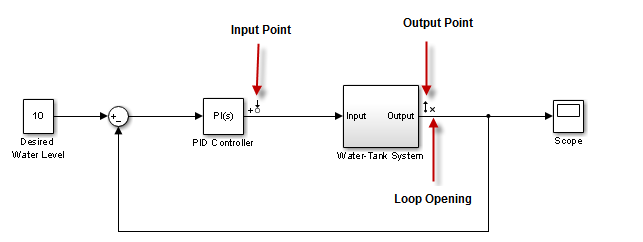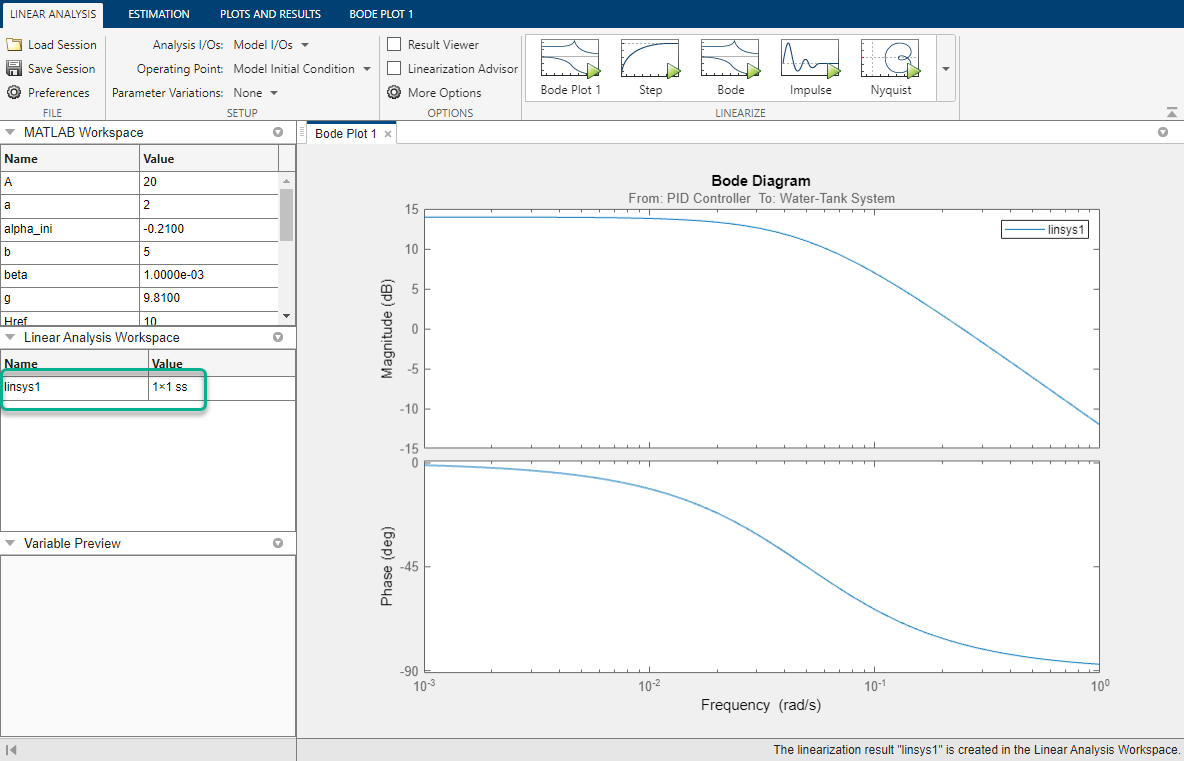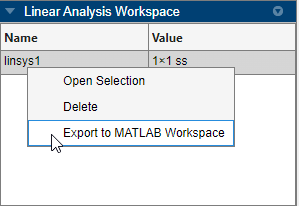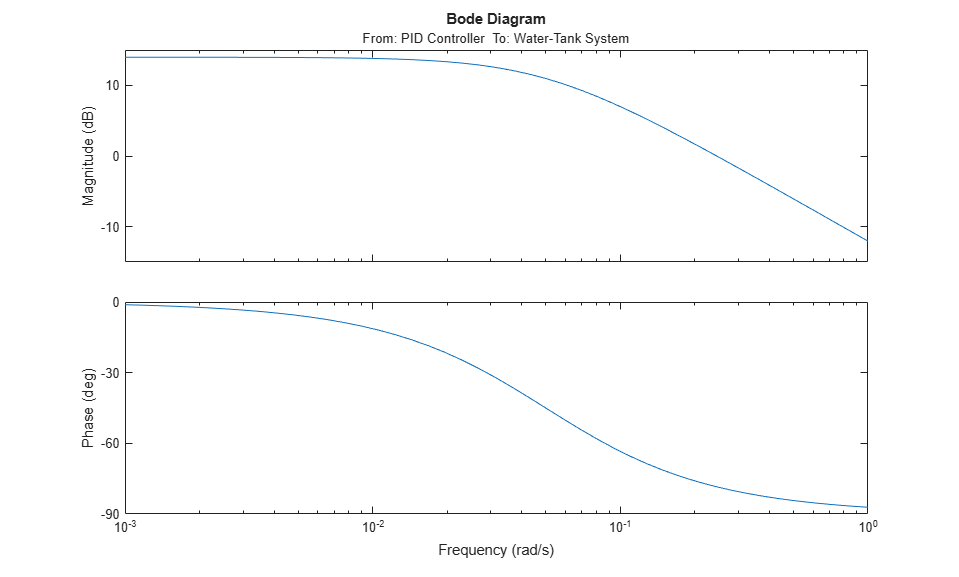Documentation

This is machine translation

Mouseover text to see original. Click the button below to return to the English version of the page.

Linearize Simulink Model at Model Operating Point

If you do not specify an operating point when linearizing a Simulink® model, the software uses the operating point specified in the model by default. The model operating point consists of the initial state and input signal values stored in the model.

For information on linearizing models at different operating points, see Linearize at Trimmed Operating Point and Linearize at Simulation Snapshot.

Linearize Simulink Model Using Linear Analysis Tool

This example shows how to linearize a Simulink model at the operating point specified in the model using the Linear Analysis Tool.

```mdl = 'watertank'; open_system(mdl)```The Water-Tank System block represents the plant in this control system and includes all of the system nonlinearities.

In the Simulink window, specify the portion of the model to linearize:

1. To specify the linearization input, right-click the output signal of the PID Controller block, and select Linear Analysis Points >Input Perturbation.

2. To specify the linearization output, right-click the output signal of the Water-Tank System, and select Linear Analysis Points >Open-loop Output. An open-loop output point is an output measurement followed by a loop opening, which removes the effects of the feedback signal on the linearization without changing the model operating point.

When you add linear analysis points, the software adds markers at their respective locations in the model. For more information on the different types of analysis points, see Specify Portion of Model to Linearize.For more information on defining analysis points in a Simulink model, see Specify Portion of Model to Linearize in Simulink Model. Alternatively, if you do not want to introduce changes to the Simulink model, you can define analysis points using the Linear Analysis Tool. For more information, see Specify Portion of Model to Linearize in Linear Analysis Tool.

To open the Linear Analysis Tool for the model, in the Simulink model window, select Analysis > Control Design > Linear Analysis.To use the analysis points you defined in the Simulink model as linearization I/Os, on the Linear Analysis tab, in the Analysis I/Os drop-down list, leave `Model I/Os` selected.

For this example, use the model operating point for linearization. In the Operating Point drop-down list, leave ```Model Initial Condition``` selected.

To linearize the system and generate a response plot for analysis, in the Linearize section, click a response. For this example, to generate a Bode plot for the resulting linear model, clickBode.

The software adds the linearized model, `linsys1`, to the Linear Analysis Workspace and generates a Bode plot for the model. `linsys1` is the linear model from the specified input to the specified output, computed at the default model operating point.For more information on analyzing linear models, see Analyze Results Using Linear Analysis Tool Response Plots.

You can also export the linearized model to the MATLAB® workspace. To do so, in the Data Browser, drag `linsys1` from the Linear Analysis Workspace to the MATLAB Workspace.Linearize Simulink Model at Command Line

This example shows how to linearize a Simulink® model at the model operating point using the `linearize` command.

```mdl = 'watertank'; open_system(mdl) ```For this system, the Water-Tank System block contains all the nonlinear dynamics. To specify the portion of the model to linearize, create an array of linearization I/O objects using the `linio` command.

Create an input perturbation analysis point at the output of the PID Controller block.

```io(1) = linio('watertank/PID Controller',1,'input'); ```

Create an open-loop output analysis point at the output of the Water-Tank System block. An open-loop output point is an output measurement followed by a loop opening, which removes the effects of the feedback signal on the linearization without changing the model operating point.

```io(2) = linio('watertank/Water-Tank System',1,'openoutput'); ```

For information on the different types of analysis points, see Specify Portion of Model to Linearize.

Linearize the model at the model operating point using the specified analysis points.

```linsys1 = linearize(mdl,io); ```

`linsys1` is the linear model from the specified input to the specified output, computed at the default model operating point.

You can then analyze the response of the linearized model. For example, plot its Bode response.

```bode(linsys1) ```For more information on analyzing linear models, see Linear Analysis (Control System Toolbox).